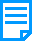Applications > Fixed Assets > Manage > Calculate Depreciation

Calculate Depreciation

Manage Calculate Depreciation allows you to calculate depreciation, update cumulative figures within Fixed Assets, and send entries to General Ledger's accumulated depreciation and depreciation expense accounts.

This Section Includes:

Calculate DepreciationThe program does not calculate gain/loss on the sale of assets. This information can be entered from the Miscellaneous tab under Asset Information (More Information). You would need to enter a manual journal entry in General Ledger to book the sale.

Depreciation is normally run on a monthly basis. If you choose to depreciate for a longer period of time you need to select the option to Adjust Straight Line to match federal tables in Company Information (More Information).

Select if you want to Run Depreciation To Book or For Tax Calculations Only. The system automatically fills in the Depreciation Thru date with the next month to be depreciated. You can change this date to a future month and year if you want to depreciate more than one month at a time or if you want to work on preparing future budgets for depreciation expenses.

Select the Calculate for Future Budgets check box if you want to calculate depreciation for a future period as if depreciation was processed for each month up to that point. Selecting this option does not allow you to post depreciation to the General Ledger since this option is designed for reporting purposes only. If this option is not selected, then depreciation is calculated to the specified Depreciation Thru date and the Finalize Post button is available to post depreciation to the General Ledger.

Depreciation Report

Click Run Report to run your depreciation and display a preview of your report. Once the preview displays you can navigate the pages, export to several formats (e.g., PDF, Excel, Word), and Print. This report displays the following information for every asset:

Tag #

Name

Capitalized Date

Depreciable Value

Net Value

Depreciation This Report

Depreciation This YearThe Depreciation This Year amount is based on your currently selected year. You can change the year in Current Financial Settings (More Information).

Totals for each Expense Account # and Accumulated Depreciation Account # are also displayed.

Tax Depreciation

When calculating depreciation for tax calculations only and with the option to calculate for future budgets deselected, you are actually depreciating the assets for tax calculation purposes. This does not post to General Ledger but it does update the 'Tax Depreciated Thru' date on the Depreciation tab of the assets.

Book Depreciation

When calculating depreciation to book and with the option to calculate for future budgets deselected, you are actually depreciating the assets and posting to General Ledger. Edit the Posting Date if desired and then click the Finalize Post button which finalizes your depreciation and post to General Ledger.

NOTE: The Post Directly to General Ledger without Editing option allows you to have your journal entry posted all the way through to the General Ledger History so that it can be viewed on reports and on the View Posted Journals page within General Ledger. This allows yo